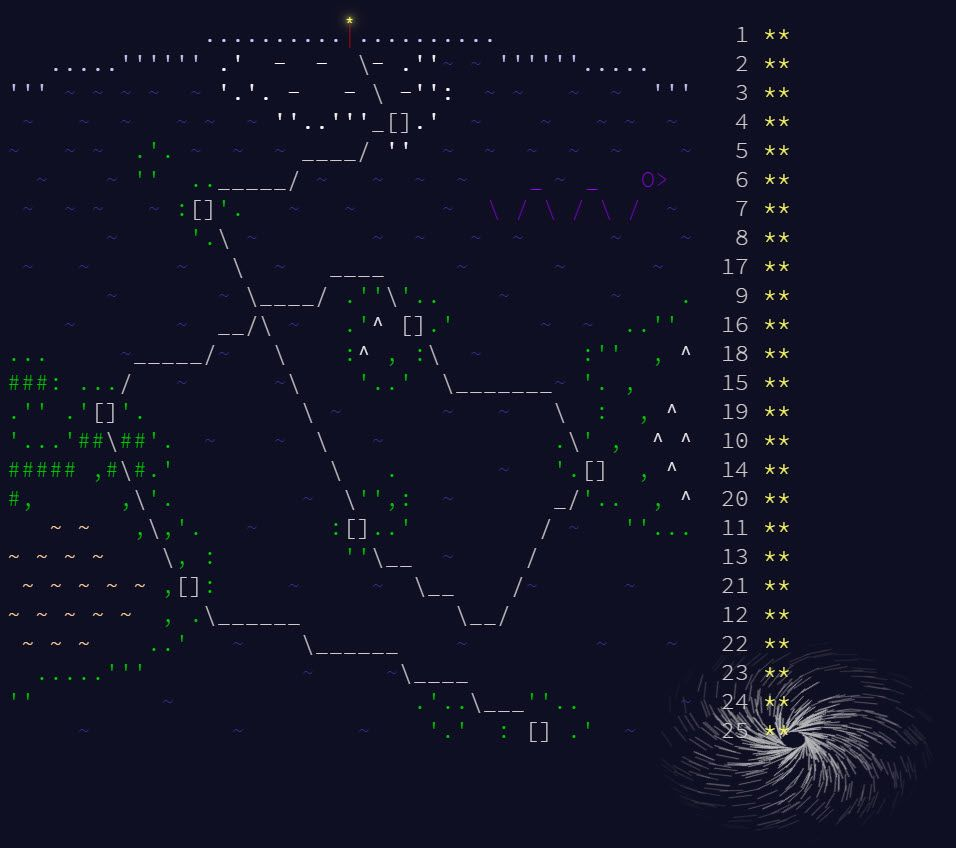It’s the end of the Advent of Code for 2020. The last week was tough - the part 1s were fairly easily doable, but part 2s often were a lot harder. In the end, I managed to solve all of this week but 1 in BaseA Alteryx. For the one I couldn’t, I used BaseA Alteryx as much as possible and the Python tool for one step.

As Christmas approaches, we all get busier, so there are less of us still going strong. I will still pick the odd alternative solution where I found ones that are sufficiently different from my own and interesting. A word of warning, some of my approaches to solving within BaseA rules are complicated and not for the faint of heart!

My previous summaries can be found:

Anyway onto the final 6 challenges.

## Day 20 -Jurassic Jigsaw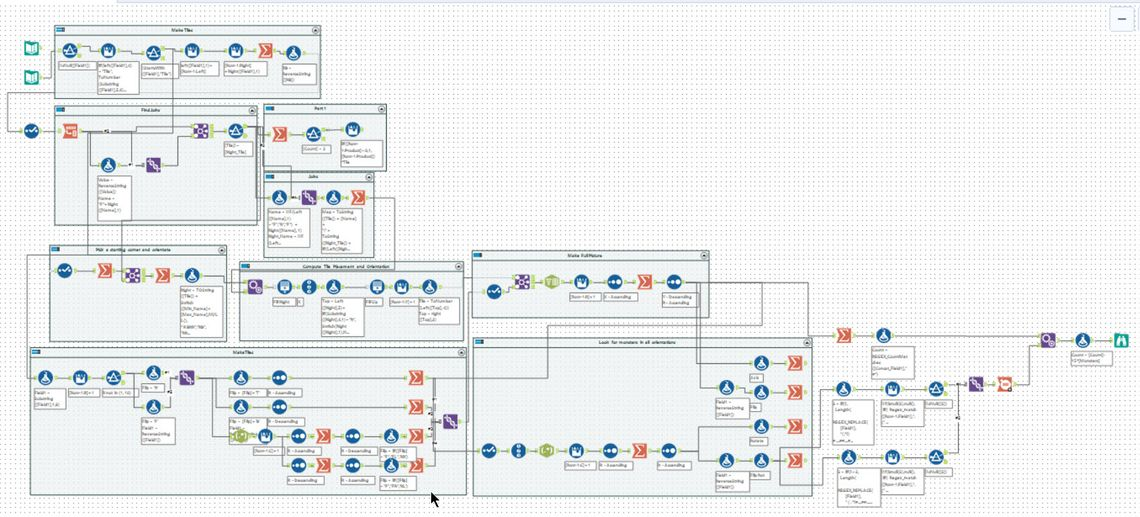Tools used: a lot - about 105, run-time: 0.8s

This puzzle was all about putting a jigsaw together. The pieces were represented with by a 10 x 10 text block with either a `#` or a `.`.

``````..##.#..#.
##..#.....
#...##..#.
####.#...#
##.##.###.
##...#.###
.#.#.#..##
..#....#..
###...#.#.
..###..###
``````

The first challenge was to find the four corners. Two pieces could fit together if a side lined up with another side of a different tile with the `#` and `.` all aligned. The second tile could be rotated or reflected. I chose to read around the 4 sides clockwise producing 4 fields (`RT`, `RR`, `RB` and `RL`). I then reversed these four strings to produce the four edges reading anti-clockwise (`FT`, `FR`, `FB` and `FL`) - note `FL` is the reverse of `RR` and `FR` is the reverse of `RL` as the tile is flipped. Next, I looked for joins with the left side being one of the `R` fields and not joining to the same cell. Finding the corners was then a case of just finding tiles with 2 possible joins.

For part 2, the first problem was putting the picture together. I chose first to pick one of the corners. Using a summarise tool, I took the lowest value tile corner. I then wanted to orientate it, so it is the bottom left tile. By looking at the two sides I had joins on, I can work out which side is the right and top in terms of the original orientation:

Side1 Side2 Top Right
RB RR RR (Right) RB (Bottom)
RR RT RT (Top) RR (Right)
RL RT RL (Left) RT (Top)
RB RL RB (Bottom) RL (Left)

I then produced a map of the joins as a single string. I did some pre-computation on this. If two tiles were both aligned the original way up then the `RR` on the first tile would join to a `FL` on the second tile; hence I need to flip `R` and `F`. The map was such that the second tile’s entry would be the opposite side to the joining side. In this example, if tile 1234 was up the original way next to 4321, the entry would be `1234RR ==> 4321RR`. I encoded all of this into a long string that looked like:

``````1907FB:2111FR 1907RB:2111RR 2017FL:3343FR 2017RL:3343RR 2477FR:3613FB 2477RR:3613RB 3671FR:2411RL ...
``````

After this, I walked right from the starting corner using a generate rows tool until I reached the end of a row - denoted by not finding another step in the map. This produced 12 rows of 1 tile. I knew the orientation for each of these tiles as knew what the right side was, so could deduce the top side and if it was reflected or not. Having found the top of all 12, a second generate rows tool allowed me to complete the tile layout, including orientation.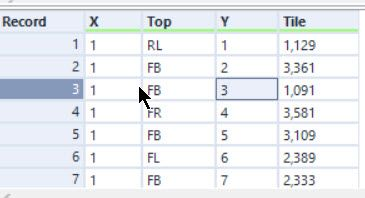I won’t go over all the remaining step in full detail as this post would be enormous but will give the rough steps instead. First, for every tile, I produced 8 copies in each of the possible rotations and reflections. This could then be joined to the layout to produce the full picture I needed, with some concatenations producing the full lines.

Within the full picture, you needed to hunt for sea monsters. I chose to search using a regular expression for the middle line (replacing spaces with `.` was all that was needed) of the sea monster. Again the picture could be in one of 8 orientations; however, you only needed to check the four rotations to determine which orientation was correct (as the monster would just be upside down). A multi-row formula allowed me to check if the previous and following rows matched for the found monster.

The second stage of this problem was fiddly, to say the least (and took a fair amount if debugging), but fun thinking it through and though the workflow is massive, it is a fairly clean solution when complete.

## Day 21 - Allergen Assessment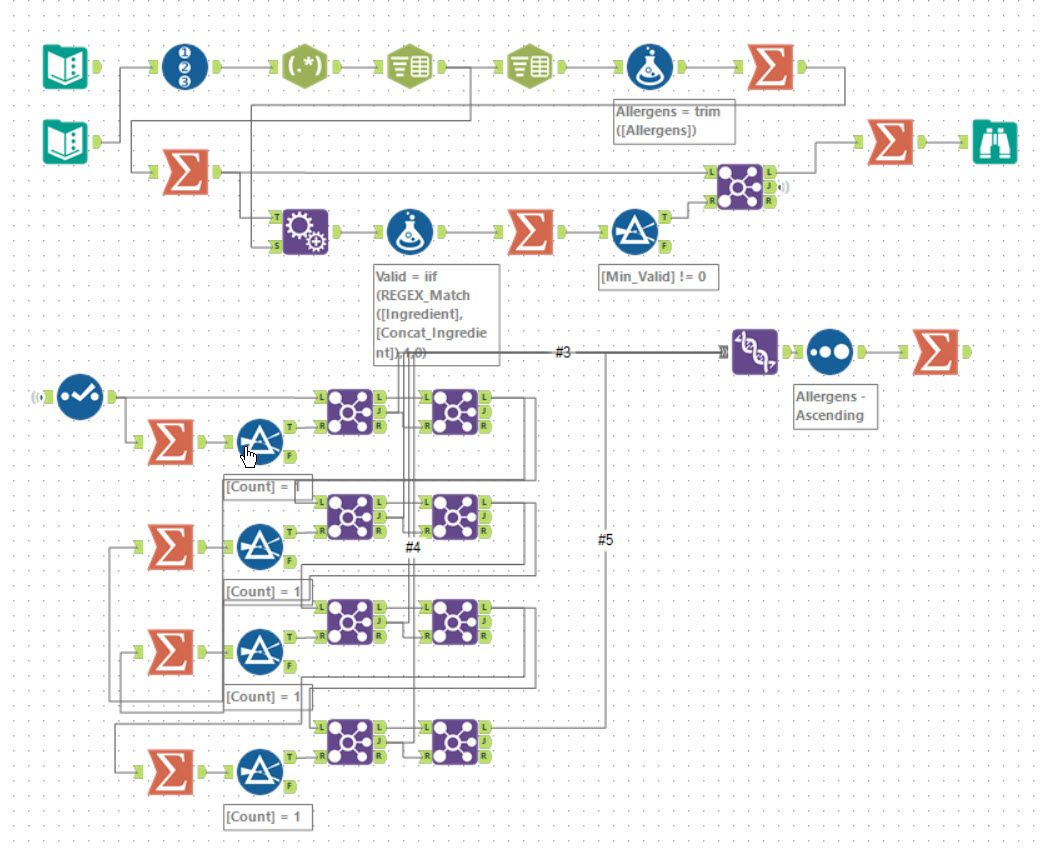Tools used: 36, run-time: 3.3s

In some ways, this was similar to some of the other days. Well suited to Alteryx with just lots of parsing and joining. Breaking it first into a list of foods with their possible allergens. For a food to be possibly an allergen, it must be present in every list for that allergen. Using an append fields to create all possible joins of lists of possible ingredient for each allergen it was then a case of removing any which were not always possible (using the `L` output of a join tool in my case).

For part 2, it was again the case of walking a hierarchy. This should probably have been an iterative macro, but as I was tired of debugging these by this point, I went for copy and pasting each block. Each row allocated the allergens which had one possible food and then removed those foods from the list and repeated. In my dataset’s case, it concluded in 4 steps (hence avoiding an iterative macro).

### More Sensible Solutions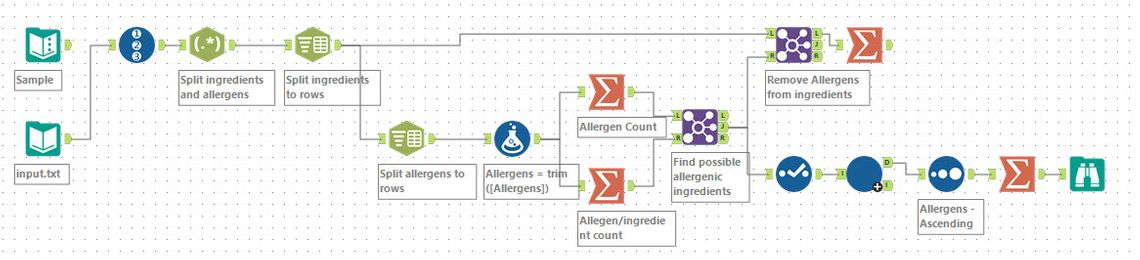I think my approach over complicated the first part somewhat. Danilang produced a much more sensible approach to solving part one. This involved just looking at the counts of allergens versus the ingredient/allergen count. It made the joins a lot cleaner to see what is going on (instead of making a dynamic regular expression as I did!).

Danilang also chose to use an iterative macro to fill all the possible rows. The iteration is as described above but involved less copy and pasting!

## Day 22 - Crab Combat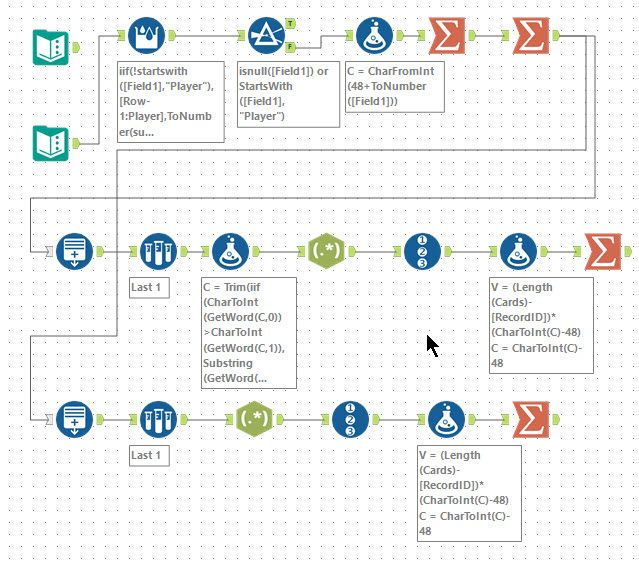Tools used: 21, run-time: 1hr 2mins

This puzzle was basically a simplified version of Top Trumps. My two children would have been pleased. Part 1 was just an iteration and could be solved with an iterative macro - however, the solution I present was built to allow me to solve part 2 (albeit very slowly!!!).

My input had a range of 1 to 50. I chose to encode these as ASCII characters so they would be 1 character each regardless of value. To make debugging easier, I chose it so that 1 mapped to character 1, 2 to 2, 3 to 3 up to 9 and then following with the next ASCII character (e.g. 10 to :). A formula of `CharFromInt(48+ToNumber([Field1]))` does this. I then joined the characters for each player into a string separated by a space. The example ends up as `92631 5847:`. Using a generate rows tool, I can iterate comparing the first letter of each word and moving around until only one word remains.

``````iif(CharToInt(GetWord(C,0))>CharToInt(GetWord(C,1)), // Player 1 wins
Substring(GetWord(C,0),1) + Left(GetWord(C,0),1) + Left(GetWord(C,1),1)
+ " " +
Substring(GetWord(C,1),1),
Substring(GetWord(C,0),1)
+ " " +
Substring(GetWord(C,1),1)+ Left(GetWord(C,1),1) + Left(GetWord(C,0),1))
``````

Because of my termination condition, I need to apply this once more to finish it (using a formula tool). Now onto part 2…

In this case, we need to keep a record of where we have been and deal with recursion! Alteryx is not designed to implement a recursive algorithm. This would clearly be pretty straight forward in a general-purpose programming language. Let’s deal with the record of past moves first and then think about recursion.

``````    // Play a turn
iif(CharToInt(GetWord(C,0))>CharToInt(GetWord(C,1)),
Substring(GetWord(C,0),1) + Left(GetWord(C,0),1) + Left(GetWord(C,1),1) + " " +Substring(GetWord(C,1),1),
Substring(GetWord(C,0),1) + " " +Substring(GetWord(C,1),1)+ Left(GetWord(C,1),1) + Left(GetWord(C,0),1))

+ " " + GetWord(C,0) + "#" + GetWord(C,1)

// Keep Old
+ Regex_Replace(C,"^[^ ]+ [^ ]+ ?", " ")
``````

This handles playing the turn and adding a new word representing the current state as `<Player1>#<Player2>` (`#` is safe to use as it’s ASCII code is less than 47). You can then look for this string in the current state string to see if you have already played this.

``````ELSEIF Contains(REGEX_Replace(C, " ! .*\$", ""),GetWord(C,0) + "#" + GetWord(C,1)) THEN
// Player 1 win by termination
``````

This gives a sequence like:

``````92631 5847:
263195 847: 92631#5847:
63195 47:82 263195#847: 92631#5847:
319564 7:82 63195#47:82 263195#847: 92631#5847:
19564 :8273 319564#7:82 63195#47:82 263195#847: 92631#5847:
``````

The next problem is how to deal with a recursive step being needed. The start of a sub-game can be easily detected by looking at the lengths of the first two words versus their first character’s ASCII code:

``````ELSEIF CharToInt(GetWord(C,0)) - 48 < Length(GetWord(C,0)) and CharToInt(GetWord(C,1))-48 < Length(GetWord(C,1)) THEN
// Do I need to recurse
Substring(GetWord(C,0),1,CharToInt(GetWord(C,0)) - 48) + " " + Substring(GetWord(C,1),1,CharToInt(GetWord(C,1))-48) + " ! "+ C
``````

When the condition is true, the process needs to recurse; 2 new words representing the starting positions of the sub-game are added at the start of the string, followed by a `!` to represent the end of the sub-game. This sub-game can then be played until a winner is found (or a new sub-game is needed).

The complicated formula’s final piece is working out how to resolve a game or sub-game when a player wins. In this case, either it ends with a single word (as per part 1) or adjusting the parent game’s state. The expression below represents player 1 winning:

``````    iif(Contains(C," ! "),
Substring(GetWord(REGEX_Replace(C, "^[^!]+ ! ", ""), 0), 1)
+ Left(GetWord(REGEX_Replace(C, "^[^!]+ ! ", ""), 0), 1)
+ Left(GetWord(REGEX_Replace(C, "^[^!]+ ! ", ""), 1), 1)
+ " " + Substring(GetWord(REGEX_Replace(C, "^[^!]+ ! ", ""), 1), 1)
+ " " + GetWord(REGEX_Replace(C, "^[^!]+ ! ", ""), 0) + "#" + GetWord(REGEX_Replace(C, "^[^!]+ ! ", ""), 1)
+ Regex_Replace(C, "^[^!]+ ! [^ ]+ [^ ]+ ?", " "),
Substring(GetWord(C,0),1) + Left(GetWord(C,0),1) + Left(GetWord(C,1),1))
``````

If the string doesn’t contain a `!` then the outer game has been completed, and a final single word is produced, and the generate rows ends. Alternatively, everything is deleted up to and including the first `!`. Then the next two words are adjusted to account for the winner of the subgame, and the state of the parent game is added to the existing string. The parent game then continues to be played.

Putting it all together gives a very long-expression, but one which will run the whole recursive game within a generate rows tool! I added a small additional step which meant an extra formula tool wasn’t needed. The long strings and hence slow manipulation make this a long process to complete, but it works in BaseA!

## Day 23 - Crab Cups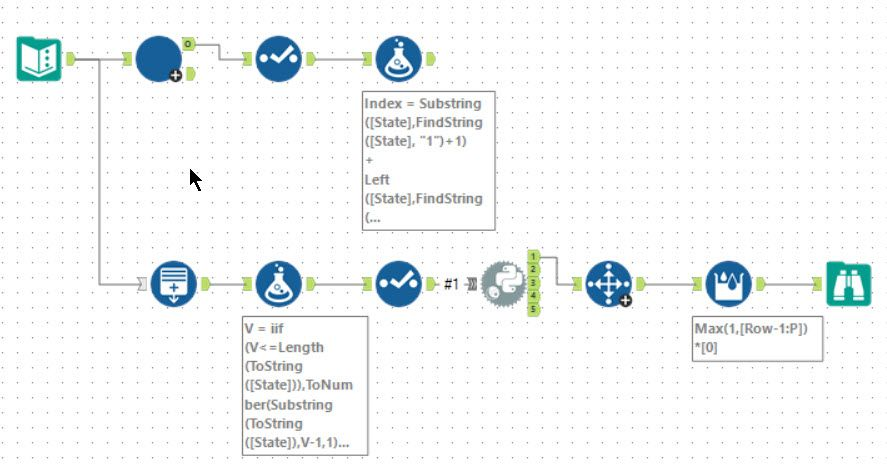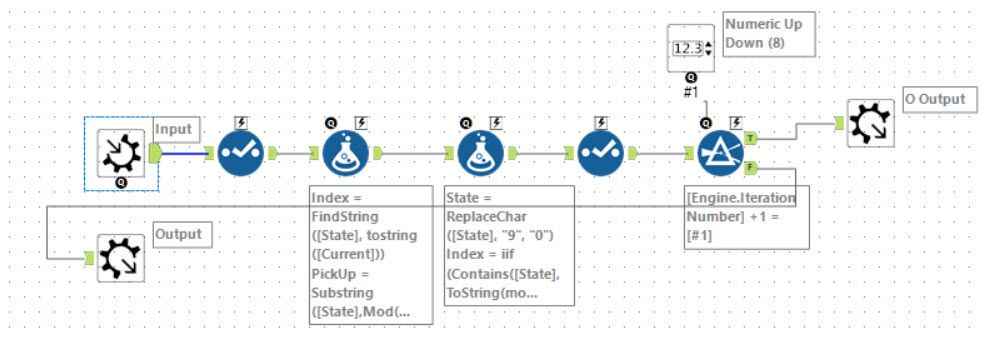Tools used: 16 (including the Python tool and iterative macro), run-time: 1min

Part 1 of this puzzle was solved using a straight forward iterative macro. Carrying a current value and the 9 number ring’s order, each iteration finds the new value and mutates the ring state. Running this 100 times produces the required answer.

For part 2, instead of a ring of 9, you need a ring of 1,000,000 numbers and to iterate it 10,000,000 times. This is impossible within BaseA rules as far as I know. I tried adding a `VarListIndexOf` function to the Abacus library which would allow the iteration to be run within a generate rows, but so far I haven’t managed to make it a reasonable solution (the lookup is an `O(n)` operation so the process is 1 with 13 zeros after it!).

I chose to solve this using the Python tool but minimising its use to just the iteration step. First, I generate the 1,000,000 rows in Alteryx and then use python to run the process. The python tool allowed me to create a linked list with a dictionary mapping value to the entry’s pointer in the linked list. This meant that the operation was O(n), so performant enough to run in a reasonable time. The code is below:

``````from ayx import Alteryx
import pandas as pd
vals = list(df['V'])
l = len(vals)

class Cup:
def __init__(self, v: int):
self.v = v
self.next = None

def __repr__(self):
return f'C({self.v}:{self.next.v})'

d = {}
for i, v in enumerate(vals):
c = Cup(v)
if i > 0:
d[vals[i - 1]].next = c

d[v] = c
d[vals[-1]].next = d[vals]

current = d[vals]

for i in range(df['games']):
c_next = current.next
current.next = current.next.next.next.next
pickup = [c_next.v, c_next.next.v, c_next.next.next.v]

i = 1
while (current.v - i if current.v - i > 0 else current.v - i + l) in pickup:
i += 1
i = (current.v - i if current.v - i > 0 else current.v - i + l)
t_next = d[i].next
d[i].next = c_next
c_next.next.next.next = t_next

current = current.next

current = d
output = []
while (current.next.v != 1):
output.append(current.v)
current = current.next

df = pd.DataFrame(output)
Alteryx.write(df,1)
``````

If I can find a way to make it work using the Abacus function, I will publish it. I’m not sure if it will be possible without specialised functions which don’t seem a valid solution.

## Day 24 - Lobby Layout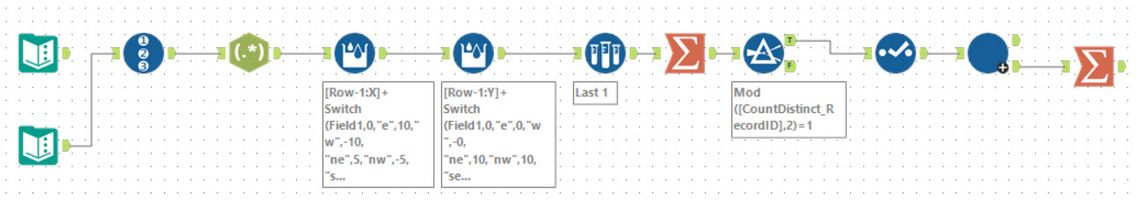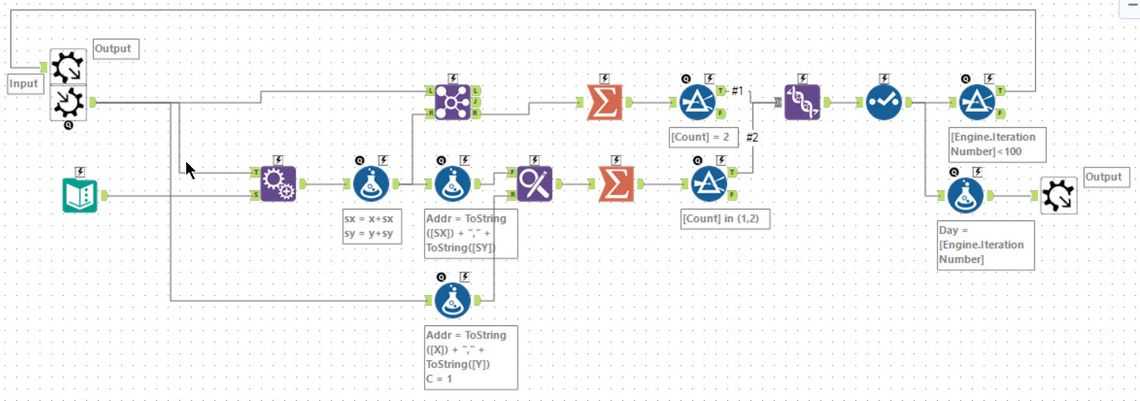Tools used: 24 (including iterative macro), run-time: 3.3s

The first challenge for today was to work out how to represent a hex-grid within Alteryx. I chose to think of it like: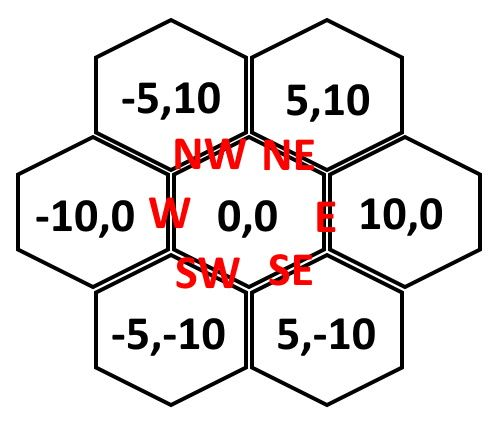It’s worth noting that this is not correct. Assuming the x co-ordinates are correct, the centres are 10 apart. The side length of the hexagon is actually then `10/√3`, so the y co-ordinate should be about 8.66. For what was needed for this puzzle, it was simpler to treat it as 10.

My solution to part one was first to parse the input string into rows using a regular expression of `ne|nw|se|sw|e|w`. I then choose to use a couple of multi-row formula tools to compute the current `x` and `y` values for each row. Finally, a sample tool picked the final cell reached.

For part 2, I chose to build an iterative macro. I passed the set of filled hexagons as the input. For each of these cells, I moved east, west, northeast, northwest, southeast and southwest (by using an append fields tool and a couple of formulas) to get all the neighbours. Having got these 6 new co-ordinates, it is then a case of joining back to the set of filled hexagons. This allows you to produce both the filled cells flipping white and the white cells becoming filled (in both cases by summing and filtering). The resulting set of filled cells can then be looped around. Using a final filter tool on `Engine.IterationNumber` allows for the macro to terminate easily.

## Day 25 - Combo Breaker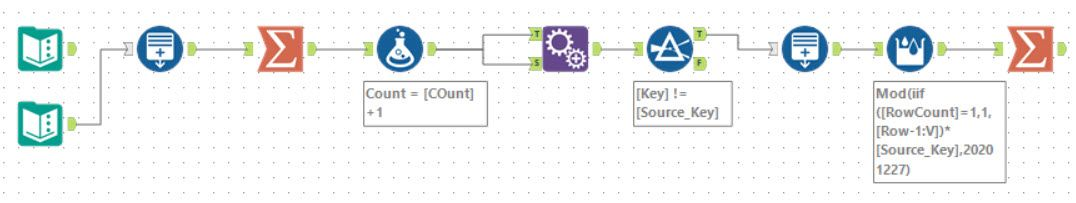Tools used: 8, run-time: 21.5s

And so we come to the final day. The first part of this was a simple generate rows tool running an iteration to work out the required number of steps. A second generate row tool then can compute the required result. A nice and gentle finish to the puzzles.

## Wrapping Up

There we have it. Advent of Code 2020 done. The table below shows my successes (* - BaseA, A - Abacus, P - Python tool):

Day 1 2 3 4 5 6 7 8 9 10 11 12 13 14 15 16 17 18 19 20 21 22 23 24 25
Part 1 * * * * * * * * * * * * * * * * * * * * * * * * *
Part 2 * * * * * * * * * * * * A * A * * * * * * * P * *

We had a few people make it to the end. I can’t comment if anyone found BaseA solutions to 15 part 2 and 23 part 2 but I believe not, some did find a workaround or pure solution using BaseA for 13 part 2. Either way 50 stars feels a great accomplishment with 47 in BaseA and all within Alteryx.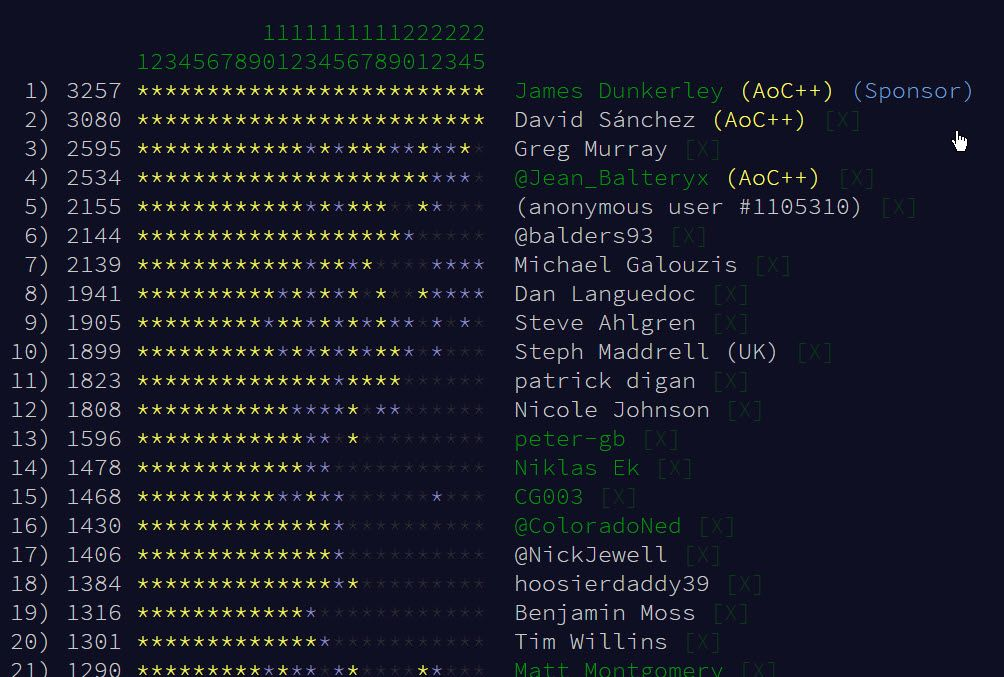The list of repositories I know of is below (no new ones added this week):

That’s a wrap for AoC 2020 - it’s been a lot of fun. Hopefully, my overviews have given you an insight into the thought processes I used to solve these puzzles and this will help you when solving your own challenges. I look forward to 2021 and the next set of challenges. A final huge thanks to Eric Wastl for setting the amazing puzzles and all the work that entails.